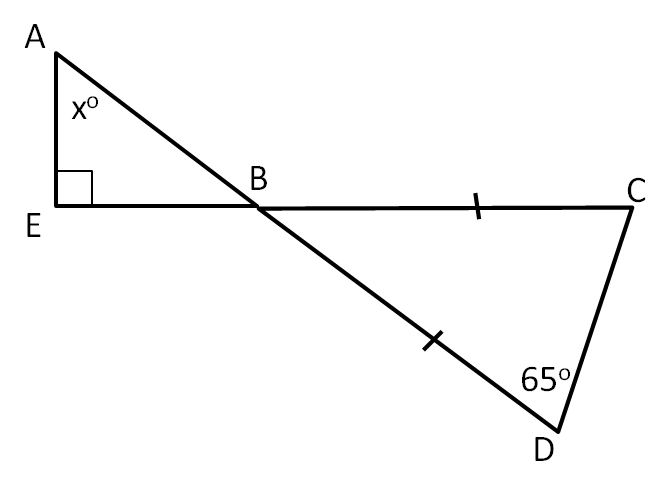#### You may also like### Isosceles

Prove that a triangle with sides of length 5, 5 and 6 has the same area as a triangle with sides of length 5, 5 and 8. Find other pairs of non-congruent isosceles triangles which have equal areas.Four rods, two of length a and two of length b, are linked to form a kite. The linkage is moveable so that the angles change. What is the maximum area of the kite?### Estimating Angles

How good are you at estimating angles?

# Shared Vertex

##### Age 11 to 14 Short Challenge Level:Triangle $BCD$ is isosceles, as $BC=BD$. Therefore, $\angle BCD = \angle BDC = 65^\circ$.

Angles in a triangle add up to $180^\circ$, so applying this in $BCD$ gives:
$\angle CBD = 180^\circ - \angle BCD - \angle BDC = 180^\circ - 65^\circ - 65^\circ = 50^\circ$.

$\angle CBD$ and $\angle ABE$ are opposite angles at $B$, so are equal. Therefore $\angle ABE = 50^\circ$.

Since the angles in triangle $ABE$ add up to $180^\circ$, $x = 180^\circ - \angle BEA - \angle ABE = 180^\circ - 90^\circ - 50^\circ = 40^\circ$.

This problem is taken from the UKMT Mathematical Challenges.
You can find more short problems, arranged by curriculum topic, in our short problems collection.